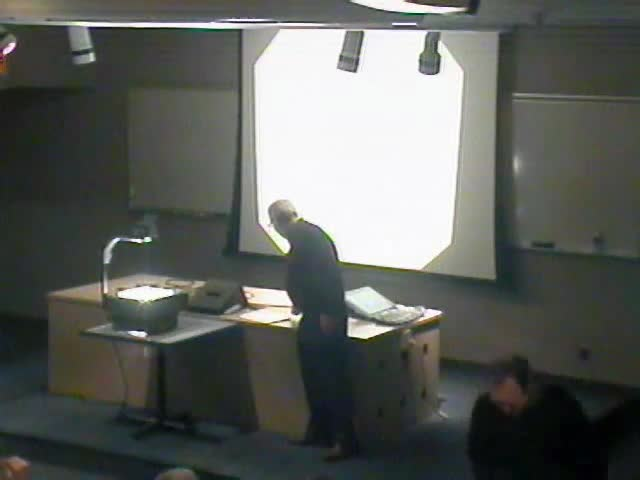### Videos

#### Travel Time Tomography, Boundary Rigidity and Electrical Impedance Tomography

##### Presenter
October 19, 2005
Keywords:
• Boundary; inverse problems
MSC:
• 31A25##### Abstract
In inverse boundary problems one attempts to determine the properties of a medium by making measurements at the boundary of the medium. In the lecture we will concentrate on two inverse boundary problems, Electrical Impedance Tomography and Travel Tomography in anisotropic media. These problems arise in medical imaging, geophysics and other fields. We will also discuss a surprising connection between these two inverse problems. Travel Time Tomography, consists in determining the index of refraction or sound speed of a medium by measuring the travel times of waves going through the medium. In differential geometry this is known as the the boundary rigidity problem. In this case the information is encoded in the boundary distance function which measures the lengths of geodesics joining points of the boundary of a compact Riemannian manifold with boundary. The inverse boundary problem consists in determining the Riemannian metric from the boundary distance function. Calderön's inverse boundary problem consists in determining the electrical conductivity inside a body by making voltage and current measurements at the boundary. This inverse problem is also called Electrical Impedance Tomography (EIT). The boundary information is Calderön's inverse boundary problem consists in determining the electrical conductivity inside a body by making voltage and current measurements at the boundary. This inverse problem is also called Electrical Impedance Tomography (EIT). The boundary information is encoded in the Dirichlet-to-Neumann (DN) map and the inverse problem is to determine the coefficients of the conductivity equation (an elliptic partial differential equation) knowing the DN map. A connection between these two inverse problems has led to a solution of the boundary rigidity problem in two dimensions for simple Riemannian metrics. We will also discuss a reconstruction method in two dimensions for the sound speed from first arrival times of waves.